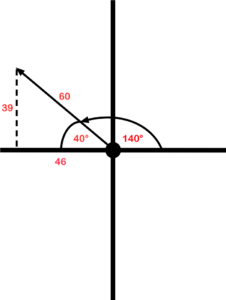# 2.3: Polar vs. Rectangular Form

$$\newcommand{\vecs}{\overset { \rightharpoonup} {\mathbf{#1}} }$$ $$\newcommand{\vecd}{\overset{-\!-\!\rightharpoonup}{\vphantom{a}\smash {#1}}}$$$$\newcommand{\id}{\mathrm{id}}$$ $$\newcommand{\Span}{\mathrm{span}}$$ $$\newcommand{\kernel}{\mathrm{null}\,}$$ $$\newcommand{\range}{\mathrm{range}\,}$$ $$\newcommand{\RealPart}{\mathrm{Re}}$$ $$\newcommand{\ImaginaryPart}{\mathrm{Im}}$$ $$\newcommand{\Argument}{\mathrm{Arg}}$$ $$\newcommand{\norm}{\| #1 \|}$$ $$\newcommand{\inner}{\langle #1, #2 \rangle}$$ $$\newcommand{\Span}{\mathrm{span}}$$ $$\newcommand{\id}{\mathrm{id}}$$ $$\newcommand{\Span}{\mathrm{span}}$$ $$\newcommand{\kernel}{\mathrm{null}\,}$$ $$\newcommand{\range}{\mathrm{range}\,}$$ $$\newcommand{\RealPart}{\mathrm{Re}}$$ $$\newcommand{\ImaginaryPart}{\mathrm{Im}}$$ $$\newcommand{\Argument}{\mathrm{Arg}}$$ $$\newcommand{\norm}{\| #1 \|}$$ $$\newcommand{\inner}{\langle #1, #2 \rangle}$$ $$\newcommand{\Span}{\mathrm{span}}$$$$\newcommand{\AA}{\unicode[.8,0]{x212B}}$$

## Polar form

When dealing with vectors, there are two ways of expressing them. Up to this point, we have used a magnitude and a direction such as 30 V @ 67°. This is what is known as the polar form. It is more often the form that we like to express vectors in.

## Rectangular form

Rectangular form breaks a vector down into X and Y coordinates. In the example below, we have a vector that, when expressed as polar, is 50 V @ 55 degrees. The first step to finding this expression is using the 50 V as the hypotenuse and the direction as the angle. Next, we draw a line straight down from the arrowhead to the X axis. What does this look like to you? If you said right triangle, give yourself a pat on the back. We then can use the angle and the hypotenuse to determine the X axis with these equations:

• cos 55°×50 = 28.7 for the X axis
• sin 55°×50 = 41 for the Y axis

This is accomplished just by transposing the ratios from what we learned previously in trigonometry.We then can express the same vector as 28.7, j 41.

## Where did that j come from?

The letter j is put in front of the y component to indicate the difference between the X and the Y. The reason j is used is this.

As a way of telling the difference between X and Y, it was decided that a letter should be put in front of the Y. The X and Y components don’t really exist, and are referred to as imaginary numbers. Because each is an imaginary number, the letter i was suggested. However, the letter i is also used as a symbol for current, so it was decided to go with the letter j instead.

## Why polarity is important

Let’s look at another example. The polar form is 60 V @ 140 degrees. This puts the vector in the second quadrant.

In the second quadrant, X is – (negative) and Y is + (positive). The angle of 140° is used from the 0° point. To use trigonometry, we need to determine what the angle is in reference to the X axis. In this example, it is 40° (the supplement of 140°). After that, we can use trigonometry to determine the X and Y components.

• cos 40°×60 = 46 for the X axis
• sin 40°×60 = 39 for the Y axis